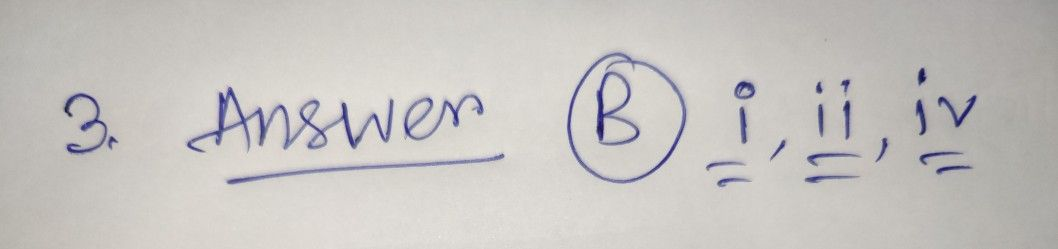Symbol
Problem$3$ Identify correct statement from the following. $\left(\right)$ $\right)$ $1$ A hypothesis is a possible answer that can be tested $4$ $n\right)$ variables are the factors that can affect the outcome of the investigation ii) Always hypothesis will be true $y\right)$ If hypothesis is incorrect test can be done with other variables $A\right)$ $2$ i, $iy$ $B\right)$ i, ii, iv C) ii, ii, $iV$ D) i, ii, i, $iy$ $4$ What do you mean by process $ski1ls7$
7th-9th grade
Biology
SolutionQanda teacher - IshitaStudent
other one
I asked easy Questions